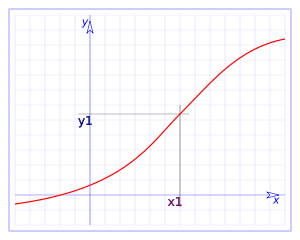# Continuity of a function at a point

There are many types of functions and forms: periodic functions defined to pieces, increasing, decreasing, hollow, convex... But between all of them, we can classify them under two more elementary sets: continuous and not continuous functions.

Commonly it is said that a function is continuous if it is possible to draw it without having need to raise the pencil of the role and therefore, to draw it with only one tracing.

Mathematically the definition is a little more elaborated.

Consider a function $$f(x)$$. We will say that it is continuous at the point $$x=a$$ if it is satisfied that the side limits of $$f(x)$$ in $$x=a$$ coincide with the value of the function at $$x=a$$: $$\displaystyle \lim_{x \to a^+} f(x)=\lim_{x \to a^-}f(x)= f(a)$$$In the following graph we observe a continuous function.and we can see that the side limits coincide with the value of the function with the point $$x1$$, $$f (x1) = y1$$. Let's see some examples: We take the function $$f(x) = e^{-x^2}$$ and let's look at the continuity of the function at the point $$x=0$$: $$\displaystyle \begin{array} {l} \lim_{x \to 0^+}f(x)=\lim_{x \to 0^+} e^{-x^2}= e^0= 1 \\ \lim_{x \to 0^-}f(x)=\lim_{x \to 0^-} e^{-x^2}= e^0= 1\end{array}$$$ and since the limits coincide with the value of the function at zero: $$f(0)=e^0=1$$, then the function is continuous at zero.

To see an example ofa function that is not continuous at a certain point, let's take the function $$\displaystyle f(x)=\left\{\begin{array}{rcl} x & \mbox{ if } & x \neq 2 \\ 0 & \mbox{ if } & x = 2\end{array}\right.$$ , and let's look at the continuity in $$x$$=2. Then we observe that: $$\displaystyle \begin{array}{l} \lim_{x \to 2^+}f(x) = \lim_{x \to 2^+} x =2 \\ \lim_{x \to 2^-}f(x)=\lim_{x \to 2^-}x=2 \end{array}$$\$ and if we evaluate the function at $$x=2$$ we have that $$f(2)=0$$, thus the function is not continuous at point $$x=2$$.